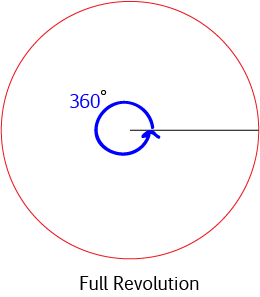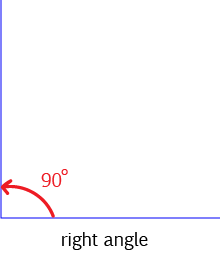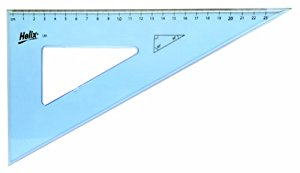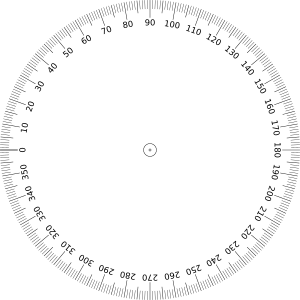Degrees (Angles)Most people measure angles in degrees. There are $360$ degrees in one full turn. Another word for a full turn is a full revolution. We use the symbol ${}^\circ$ for degrees, so we would write this as $360^\circ$.

Mathematicians really prefer to work with angles measured in radians, but when we start out, we learn about degrees. If you would like to learn more about radians, and why mathematiciansread prefer them, read the article on measuring angles in radians. Surprisingly enough, it isn't because they want to make mathematics inaccessible to all but a select few. Things just work out better with radians.

What Does One Degree Look Like?

One degree is a teeny little angle that you almost wouldn't notice. There is a $1^\circ$ angle between the two lines in the picture below.The Sizes of Some other Common AnglesA full turn (a full revolution) is $360^\circ$. This is also the angle at the centre of a whole circle.A straight angle (a half turn) is $180^\circ$. This is also the angle made by the two ends of a straight line segment, and the angle at the centre of a semicircle.A right angle (also known as a quarter turn) is $90^\circ$. This is also the angle at the centre of a quarter circle.

How Do We Measure Angles?We can measure some angles using a set square like the one in the picture. This set square will let you measure angles of $30^\circ, 60^\circ$ and $90^\circ$. Other set squares will let you measure angles of $45^\circ$ and $90^\circ$.

We can measure many angles using a protractor like the one below:Place the bottom of the protractor on one arm of the angle, and the other arm of the angle will line up with one of the markings on the outside of the protractor to tell you how many degrees there are in the angle.

The protractor above measures angles from $0^\circ$ to $180^\circ$. There are other protractors that are full circles and are designed to measure angles from $0^\circ$, all the way up to $360^\circ$ like the one below.I have never really understood the point of these. I find it much easier to use the semi-circular protractor.

Who Decided That There Should Be $360^\circ$ in a Full Rotation?

This is a really good question, and I don't think anyone is really sure. There are a number of theories, however.

According to one theory, ancient astronomers noticed that the Sun seems to move forward in its path along the ecliptic by about 1 degree every day. As the ancient Persian calendar used a year with 360 days and these astronomers were Persian, they divided the circle up into 360 equal parts, one for each day. These parts became the degrees that we use to measure angles.

Another theory says that the Babylonians, who, incidentally, loved the number $60$, divided the circle up using equilateral triangles. Each angle in an equilateral triangle is $60^\circ$. The Babylonians divided the angles of the equilateral triangle up into $60$ equal parts to match their sexagesimal number system. Each equal part was $1$ degree, and the circle was divided into $6$ equilateral triangles, and hence $360^\circ$.

The Ancient Greeks also divided a circle up into $360^\circ$. It has been suggested that they borrowed this idea from the Babylonians. However, Eratosthenes (the guy with the sieve for finding prime numbers) made a simpler subdivision of the circle into $60$ equal parts.

The Ancient Indians also divided a circle into $360$ equal parts, but we don't know why.

Finally, $360$ is a wonderful number to split something into because it is divisible by so many other numbers. This probably was a factor in the decision (sorry, couldn't resist the bad maths joke).

It is a likely that more than one of these reasons contributed to the decision to divide the circle up into $360^\circ$. I guess we'll never know for sure, but I think this is very likely.

Description

In this mini book, you will learn about

1. Alternate angles
2. Cointerior angles
4. Vertically Opposite Angles

and several other topics related to lines and angles.

Audience

Year 10 or higher

Learning Objectives

To learn the basics of Lines and Angles stream of Geometry

Author: Subject Coach